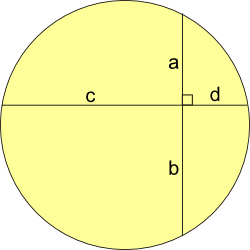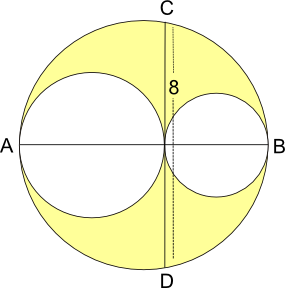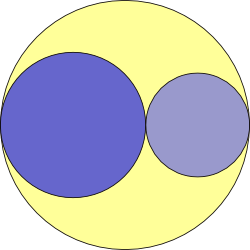#### You may also like### Fitting In

The largest square which fits into a circle is ABCD and EFGH is a square with G and H on the line CD and E and F on the circumference of the circle. Show that AB = 5EF. Similarly the largest equilateral triangle which fits into a circle is LMN and PQR is an equilateral triangle with P and Q on the line LM and R on the circumference of the circle. Show that LM = 3PQ### Look Before You Leap

Can you spot a cunning way to work out the missing length?### Triangle Midpoints

You are only given the three midpoints of the sides of a triangle. How can you construct the original triangle?

# Partly Circles

##### Age 14 to 16Challenge Level

Here are three problems involving circles.

Can you solve them?
Can you find relationships between the three problems?

Firstly Show that ab = cd (where a, b, c, d are lengths)Secondly:

These three circles are drawn so that they touch each other, and their centres are all on the line AB.

If CD is $8$ units in length, what is the area of the region shaded yellow?Lastly: If the area shaded yellow is equal to the area of the larger of the two blue circles, what is the relationship between the radii of the three circles?